# 2 Introduction to SPsimSeq

SPsimSeq is a semi-parametric simulation procedure for simulating bulk and single-cell RNA-seq data. It is designed to maximally retain the characteristics of real RNA-seq data with reasonable flexibility to simulate a wide range of scenarios. In a first step, the logarithmic counts per millions of reads (log-CPM) values from a given real dataset are used for semi-parametrically estimating gene-wise distributions and the between-genes correlation structure. In particular, the estimation of the probability distributions uses the fast log-linear model-based density estimation approach developed by Efron and Tibshirani (1996) and Lindsey (1974). The method makes use of the Gaussian-copulas (Cario and Nelson 1997) to retain the between-genes correlation structure, as implemented by Hawinkel et al. (2019) for microbiome data simulation. Arbitrarily large datasets, with realistically varying library sizes, can be sampled from these distributions while maintaining the correlation structure between the genes. Our method has an additional step to explicitly account for the high abundance of zero counts, typical for single-cell RNA-seq data. This step models the probability of zero counts as a function of the mean expression of the gene and the library size (read depth) of the cell (both in log scale). Zero counts are then added to the simulated data such that the observed relationship (zero probability to mean expression and library size) is maintained. In addition, our method simulates DE by separately estimating the distributions of the gene expression from the different populations (for example treatment groups) in the source data, and subsequently sampling a new dataset from each group. The details of the SPsimSeq procedures, implementations and benchmarking results can be found in the supplementary file.

In this documentation, we will demonstrate SPsimSeq for simulating bulk and single-cell RNA-seq, data subsequently compare the characteristics of the simulated data with the real source data.

# 3 Installing SPsimSeq

The package can be installed and loaded using the following commands:

## Install SPsimSeq
library(devtools)
install_github("CenterForStatistics-UGent/SPsimSeq")

or from BioConductor

## Install SPsimSeq
library(BiocManager)
BiocManager::install("SPsimSeq")
# load package
library(SPsimSeq) 
## 

# 4 Demonstration

## 4.1 Example 1: simulating bulk RNA-seq

Zhang RNA-seq data (Zhang et al. 2015): The data contains 498 neuroblastoma tumors. In short, unstranded poly(A)+ RNA sequencing was performed on the HiSeq 2000 instrument (Illumina). Paired-end reads with a length of 100 nucleotides were obtained. To quantify the full transcriptome, raw fastq files were processed with Kallisto v0.42.4 (index build with GRCh38-Ensembl v85). The pseudo-alignment tool Kallisto (???) was chosen above other quantification methods as it is performing equally good but faster. For this study, a subset of 172 tumors (samples) with high-risk disease were selected, forming two groups: the MYCN amplified ($$n_1$$ = 91) and MYCN non-amplified ($$n_2$$ = 81) tumours as used in (???). Sometimes we refer this dataset to us the Zhang data or the Zhang neuroblastoma data. A subset of this dataset (5000 randomly selected genes) is available with the SPsimSeq package for illustration purpose only.

 # load the Zhang bulk RNA-seq data (availabl with the package)
data("zhang.data.sub")
# filter genes with sufficient expression (important step to avoid bugs)
zhang.counts <- zhang.data.sub$counts MYCN.status <- zhang.data.sub$MYCN.status #The grouping variable

This dataset is now used as a template for semiparametric data generation. We simulate only a single data (n.sim = 1) with the following properties: - 3000 genes ( n.genes = 3000) - 172 samples (tot.samples = 172) – equal to the source data - the samples are equally divided into 2 groups each with 90 samples (group.config = c(0.5, 0.5)) – almost equal to the source data - all samples are from a single batch (batch.config = 1) - we add 10% DE genes (pDE = 0.1) - the DE genes have a log-fold-change of at least 0.5 in the source data (lfc.thrld = 0.5) - we do not model the zeroes separately, they are the part of density estimation (model.zero.prob = FALSE)

 set.seed(6452) #Set seed for reproducibility
# simulate data
sim.data.bulk <- SPsimSeq(n.sim = 1, s.data = zhang.counts,
group = MYCN.status, n.genes = 3000, batch.config = 1,
group.config = c(0.5, 0.5), tot.samples = ncol(zhang.counts),
pDE = 0.1, lfc.thrld = 0.5, result.format = "list", return.details = TRUE)
## Estimating featurewise correlations ...
## Selecting candidate DE genes ...
## Note: The number of null genes (not DE) in the source data is 968 and the number of null genes required to be included in the simulated data is 2700. Therefore, candidiate null genes are sampled with replacement.
## Note: The number of DE genes detected in the source data is 32 and the number of DE genes required to be included in the simulated data is 300. Therefore, candidiate DE genes are sampled with replacement.
## Estimating densities ...
## Configuring design ...
## Simulating data ...
##  ...1 of 1

Next, we explore the data we just generated.

 sim.data.bulk1 <- sim.data.bulk$sim.data.list[] head(sim.data.bulk1$counts[, seq_len(5)])  # count data
##        Sample_1 Sample_2 Sample_3 Sample_4 Sample_5
## Gene_1       12        1        4       25       64
## Gene_2        0        7        0        4        1
## Gene_3        5        0        2        0        2
## Gene_4        4        1        0        0        2
## Gene_5        4        1        0        9        7
## Gene_6        3        0        3        0        0
 head(sim.data.bulk1$colData) # sample info ## Batch Group sim.Lib.Size ## Sample_1 1 1 762054 ## Sample_2 1 1 665409 ## Sample_3 1 1 727419 ## Sample_4 1 1 385784 ## Sample_5 1 1 495890 ## Sample_6 1 1 774708  head(sim.data.bulk1$rowData)        # gene info
##        DE.ind     source.ID
## Gene_2   TRUE RP11-1084I9.1
## Gene_3   TRUE  RP11-44F14.8
## Gene_4   TRUE RP11-662J14.2
## Gene_5   TRUE  RP11-326L2.1
## Gene_6   TRUE   RP5-998H6.2

Since we set return.details = TRUE, we have access to all density estimates, which can be extracted with the evaluateDensities function.

geneDens = evaluateDensities(sim.data.bulk, newData = rownames(zhang.counts))
#This returns for every sample, the midpoints (mids) and associated densities (gy)

Next we compare the data generated with SPsimSeq with the original data properties to show that they are realistic and close to the real data.

# compare the distributions of the mean expressions, variability,
# and fraction of zero counts per gene
library(LSD) # for generating heatmap plots
# normalize counts for comparison
Y0.log.cpm <- log2(edgeR::cpm(zhang.counts)+1)
Y1.log.cpm <- log2(edgeR::cpm(sim.data.bulk1$counts)+1) Y0.log.cpm <- Y0.log.cpm[rowMeans(Y0.log.cpm>0)>=0.1, ] Y1.log.cpm <- Y1.log.cpm[rowMeans(Y1.log.cpm>0)>=0.1, ] rowVars <- function(X){apply(X, 1, var, na.rm=TRUE)} rowCVs <- function(X){apply(X, 1, function(x) sd(x, na.rm=TRUE)/mean(x, na.rm=TRUE))} par(mfrow=c(1, 3)) boxplot(list(real.data=log(colSums(zhang.counts)), simulated.data=log(sim.data.bulk1$colData$sim.Lib.Size)), main="library size") boxplot(list(real.data=rowMeans(Y0.log.cpm), simulated.data=rowMeans(Y1.log.cpm)), main="mean expression of genes") boxplot(list(real.data=rowVars(Y0.log.cpm), simulated.data=rowVars(Y1.log.cpm)), main="variance of gene expressions")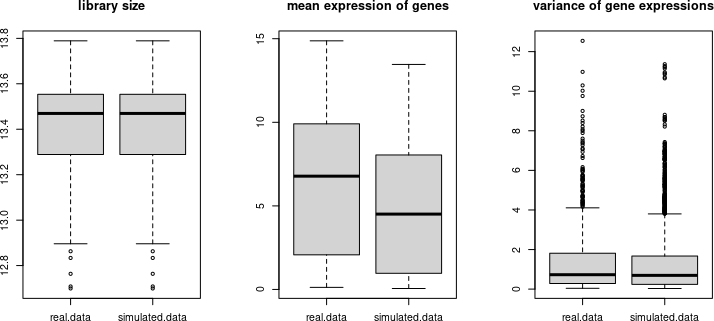The library sizes are identical since they were not modelled (see variable.lib.size argument in ?SPsimSeq). Next, we look at mean-variance trends # compare the relationship between the mean and variability par(mfrow=c(1,3), mar=c(4,4,4,1)) heatscatter(rowMeans(Y0.log.cpm), rowCVs(Y0.log.cpm), ylim=c(0, 6), xlim=c(0, 16), colpal="bl2gr2rd", main="real data", xlab="mean log2-CPM", ylab="coefficients of variation", cexplot=0.5, alpha = 60, cex.lab=1.25) heatscatter(rowMeans(Y1.log.cpm), rowCVs(Y1.log.cpm), ylim=c(0, 6), xlim=c(0, 16), main="SPsimSeq", xlab="mean log2-CPM", ylab="coefficients of variation", cexplot=0.5, alpha = 60, colpal="bl2gr2rd", cex.lab=1.25) n.gride <- 1000 min.g <- seq(0, 20, length.out = n.gride+1)[-n.gride] max.g <- seq(0, 20, length.out = n.gride+1)[-1] mid.g <- (min.g+max.g)/2 f.real <- vapply(seq_len(n.gride), FUN.VALUE = double(1), function(r){ x <- Y0.log.cpm[rowMeans(Y0.log.cpm)<=max.g[r] & rowMeans(Y0.log.cpm)>min.g[r],] y <- ifelse(!is.null(dim(x)), mean(rowCVs(x)), mean(sd(x)/mean(x))) y }) f.SPsim <- vapply(seq_len(n.gride), FUN.VALUE = double(1), function(r){ x <- Y1.log.cpm[rowMeans(Y1.log.cpm)<=max.g[r] & rowMeans(Y1.log.cpm)>min.g[r],] y <- ifelse(!is.null(dim(x)), mean(rowCVs(x)), mean(sd(x)/mean(x))) y }) sm1 <- loess(I(f.SPsim-f.real)~mid.g) plot(mid.g, f.SPsim-f.real, xlim=c(0, 14), col="lightskyblue", pch=20, cex.lab=1.25, cex.main=1.4, main="SPsimSeq - real data", ylab="difference", xlab="mean log2-CPM") lines(mid.g,predict(sm1, newdata = mid.g), col="blue", lwd=3)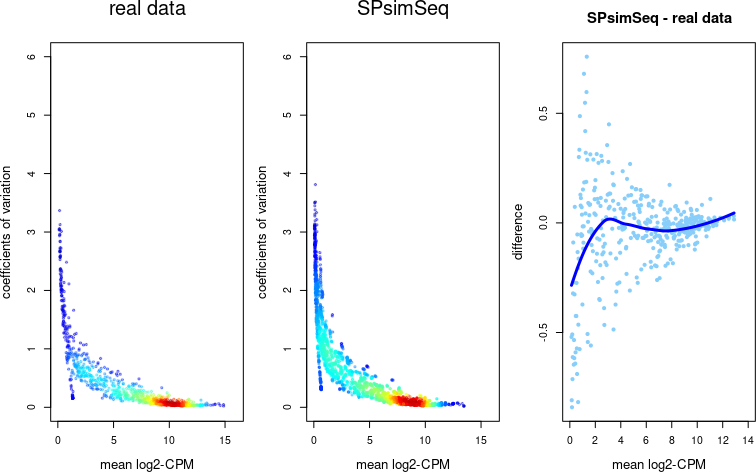We emulated the correlation networks found in the real data (see genewiseCor argument). Now we check if the correlation patterns in the synthetic data resemble those in the real data. # compare the correlation between genes and samples cor.mat.Y0 <- cor(t(Y0.log.cpm)) cor.mat.Y1 <- cor(t(Y1.log.cpm)) cor.vec.Y0 <- cor.mat.Y0[upper.tri(cor.mat.Y0)] cor.vec.Y1 <- cor.mat.Y1[upper.tri(cor.mat.Y1)] par(mfrow=c(1,3), mar=c(4,4,3.5,1)) hist(cor.vec.Y0, nclass = 30, probability = TRUE, border="gray", col="steelblue1", main="real data", xlab="Genewise correlations", ylim=c(0, 3.5), xlim=c(-1, 1), cex.lab=1.25) hist(cor.vec.Y1, nclass = 30, probability = TRUE, border="gray", col="steelblue1", main="SPsimSeq", xlab="Genewise correlations", ylim=c(0, 3.5), xlim=c(-1, 1), cex.lab=1.25) plot(seq(-1, 1, 0.1), seq(-1, 1, 0.1), type="n", xlab="quantile (real data)", ylab="quantile (simulated data)", main="correlation quantile-quantile plot") abline(0, 1, col="gray") points(quantile(cor.vec.Y0, seq(0, 1, 0.001)), quantile(cor.vec.Y1, seq(0, 1, 0.001)), col="blue", pch=20, cex=1.5, cex.lab=1.25)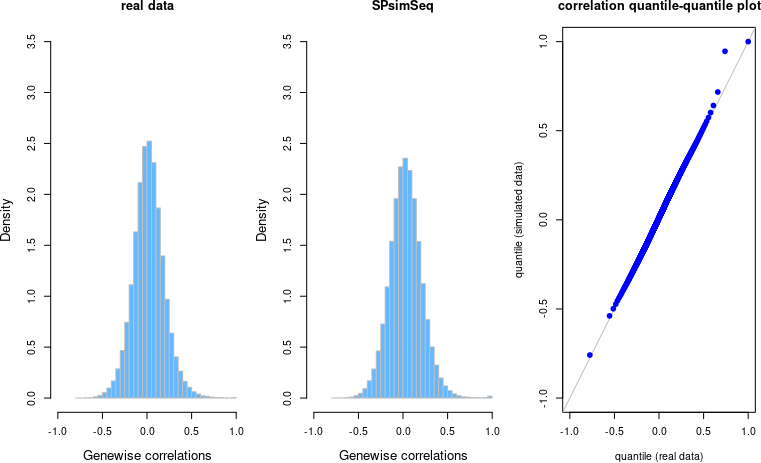## 4.2 Example 2: simulating single-cell RNA-seq (containing read-counts) Neuroblastoma NGP cells scRNA-seq data (NGP data) retrieved from (Verboom et al. 2019) (GEO accession GSE119984): This dataset is generated for a cellular perturbation experiment on the C1 instrument (SMARTer protocol) (Verboom et al. 2019). This total RNA-seq dataset contains 83 NGP neuroblastoma cells, of which 31 were treated with 8$$\mu$$M of nutlin-3 and the other 52 cells were treated with vehicle (controls). In the subsequent sections, this dataset is referred to us the NGP single-cell RNA-seq data. We simulate only a single scRNA-seq data (n.sim = 1) with the following property - 4000 genes (n.genes = 4000) - 100 cells (tot.samples = 100) - the cells are equally divided into 2 groups each with 50 cells (group.config = c(0.5, 0.5)) - all cells are from a single batch (batch.config = 1) - we add 10% DE genes (pDE = 0.1) - the DE genes have a log-fold-change of at least 0.5 - we model the zeroes separately (model.zero.prob = TRUE) - the ouput will be in SingleCellExperiment class object (result.format = “SCE”) library(SingleCellExperiment) ## Loading required package: SummarizedExperiment ## Loading required package: MatrixGenerics ## Loading required package: matrixStats ## ## Attaching package: 'matrixStats' ## The following object is masked _by_ '.GlobalEnv': ## ## rowVars ## ## Attaching package: 'MatrixGenerics' ## The following object is masked _by_ '.GlobalEnv': ## ## rowVars ## The following objects are masked from 'package:matrixStats': ## ## colAlls, colAnyNAs, colAnys, colAvgsPerRowSet, colCollapse, ## colCounts, colCummaxs, colCummins, colCumprods, colCumsums, ## colDiffs, colIQRDiffs, colIQRs, colLogSumExps, colMadDiffs, ## colMads, colMaxs, colMeans2, colMedians, colMins, colOrderStats, ## colProds, colQuantiles, colRanges, colRanks, colSdDiffs, colSds, ## colSums2, colTabulates, colVarDiffs, colVars, colWeightedMads, ## colWeightedMeans, colWeightedMedians, colWeightedSds, ## colWeightedVars, rowAlls, rowAnyNAs, rowAnys, rowAvgsPerColSet, ## rowCollapse, rowCounts, rowCummaxs, rowCummins, rowCumprods, ## rowCumsums, rowDiffs, rowIQRDiffs, rowIQRs, rowLogSumExps, ## rowMadDiffs, rowMads, rowMaxs, rowMeans2, rowMedians, rowMins, ## rowOrderStats, rowProds, rowQuantiles, rowRanges, rowRanks, ## rowSdDiffs, rowSds, rowSums2, rowTabulates, rowVarDiffs, rowVars, ## rowWeightedMads, rowWeightedMeans, rowWeightedMedians, ## rowWeightedSds, rowWeightedVars ## Loading required package: GenomicRanges ## Loading required package: stats4 ## Loading required package: BiocGenerics ## ## Attaching package: 'BiocGenerics' ## The following objects are masked from 'package:stats': ## ## IQR, mad, sd, var, xtabs ## The following objects are masked from 'package:base': ## ## Filter, Find, Map, Position, Reduce, anyDuplicated, append, ## as.data.frame, basename, cbind, colnames, dirname, do.call, ## duplicated, eval, evalq, get, grep, grepl, intersect, is.unsorted, ## lapply, mapply, match, mget, order, paste, pmax, pmax.int, pmin, ## pmin.int, rank, rbind, rownames, sapply, setdiff, sort, table, ## tapply, union, unique, unsplit, which.max, which.min ## Loading required package: S4Vectors ## ## Attaching package: 'S4Vectors' ## The following objects are masked from 'package:base': ## ## I, expand.grid, unname ## Loading required package: IRanges ## Loading required package: GenomeInfoDb ## Loading required package: Biobase ## Welcome to Bioconductor ## ## Vignettes contain introductory material; view with ## 'browseVignettes()'. To cite Bioconductor, see ## 'citation("Biobase")', and for packages 'citation("pkgname")'. ## ## Attaching package: 'Biobase' ## The following object is masked from 'package:MatrixGenerics': ## ## rowMedians ## The following objects are masked from 'package:matrixStats': ## ## anyMissing, rowMedians # load the NGP nutlin data (availabl with the package, processed with SMARTer/C1 protocol, and contains read-counts) data("scNGP.data") set.seed(654321) # simulate data (we simulate here only a single data, n.sim = 1) sim.data.sc <- SPsimSeq(n.sim = 1, s.data = scNGP.data, group = scNGP.data$characteristics..treatment,
n.genes = 4000, batch.config = 1,
group.config = c(0.5, 0.5), tot.samples = 100,
pDE = 0.1, lfc.thrld = 0.5, model.zero.prob = TRUE,
result.format = "SCE")
## Estimating featurewise correlations ...
## Selecting candidate DE genes ...
## Note: The number of null genes (not DE) in the source data is 981 and the number of null genes required to be included in the simulated data is 3600. Therefore, candidiate null genes are sampled with replacement.
## Note: The number of DE genes detected in the source data is 19 and the number of DE genes required to be included in the simulated data is 400. Therefore, candidiate DE genes are sampled with replacement.
## Fitting zero probability model ...
## Estimating densities ...
## Configuring design ...
## Simulating data ...
##  ...1 of 1

Take a quick peek at the data.

 sim.data.sc1 <- sim.data.sc[]
class(sim.data.sc1)
##  "SingleCellExperiment"
## attr(,"package")
##  "SingleCellExperiment"
 head(counts(sim.data.sc1)[, seq_len(5)])
##        Sample_1 Sample_2 Sample_3 Sample_4 Sample_5
## Gene_1        0       25       47       25        0
## Gene_2       77      381       14      609      184
## Gene_3        0        0        0       37        0
## Gene_4        0       35        0        0        0
## Gene_5       18        0       52       27        8
## Gene_6        7       24       85        0       12
 colData(sim.data.sc1)
## DataFrame with 100 rows and 3 columns
##                  Batch       Group sim.Lib.Size
##            <character> <character>    <numeric>
## Sample_1             1     vehicle         6842
## Sample_2             1     vehicle        31644
## Sample_3             1     vehicle        29766
## Sample_4             1     vehicle        34072
## Sample_5             1     vehicle        19042
## ...                ...         ...          ...
## Sample_96            1      nutlin        41566
## Sample_97            1      nutlin        36668
## Sample_98            1      nutlin        19377
## Sample_99            1      nutlin        33645
## Sample_100           1      nutlin        21125
 rowData(sim.data.sc1)
## DataFrame with 4000 rows and 2 columns
##              DE.ind       source.ID
##           <logical>     <character>
## Gene_1         TRUE ENSG00000105613
## Gene_2         TRUE ENSG00000166508
## Gene_3         TRUE ENSG00000119946
## Gene_4         TRUE ENSG00000164306
## Gene_5         TRUE ENSG00000105613
## ...             ...             ...
## Gene_3996     FALSE ENSG00000101608
## Gene_3997     FALSE ENSG00000239335
## Gene_3998     FALSE ENSG00000100890
## Gene_3999     FALSE ENSG00000213171
## Gene_4000     FALSE ENSG00000125386

Look at basic data properties.

# normalize counts for comparison
Y0.log.cpm  <- log2(edgeR::cpm(counts(scNGP.data))+1)
Y1.log.cpm  <- log2(edgeR::cpm(counts(sim.data.sc1))+1)
Y0.log.cpm  <- Y0.log.cpm[rowMeans(Y0.log.cpm>0)>=0.1, ]
Y1.log.cpm  <- Y1.log.cpm[rowMeans(Y1.log.cpm>0)>=0.1, ]
rowVars <- function(X){apply(X, 1, var, na.rm=TRUE)}
rowCVs <- function(X){apply(X, 1, function(x) sd(x, na.rm=TRUE)/mean(x, na.rm=TRUE))}
rowZeroFrac <- function(X){apply(X, 1, function(x) mean(x==0, na.rm=TRUE))}
par(mfrow=c(1, 3))
boxplot(list(real.data=colSums(counts(scNGP.data)),
simulated.data=colData(sim.data.sc1)\$sim.Lib.Size),
main="library size")
boxplot(list(real.data=rowMeans(Y0.log.cpm),
simulated.data=rowMeans(Y1.log.cpm)),
main="mean expression of genes")
boxplot(list(real.data=rowVars(Y0.log.cpm),
simulated.data=rowVars(Y1.log.cpm)),
main="variance of gene expressions")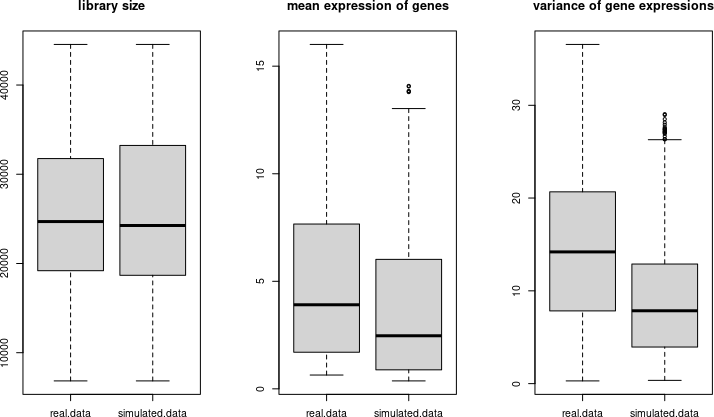Compare mean-variance distributions

# compare the relationship between the mean and variability
par(mfrow=c(1,3), mar=c(4,4,4,1))
heatscatter(rowMeans(Y0.log.cpm), rowCVs(Y0.log.cpm), ylim=c(0, 6), xlim=c(0, 16),
colpal="bl2gr2rd", main="real data", xlab="mean log2-CPM",
ylab="coefficients of variation", cexplot=0.5, alpha = 60, cex.lab=1.25)
heatscatter(rowMeans(Y1.log.cpm), rowCVs(Y1.log.cpm), ylim=c(0, 6), xlim=c(0, 16),
main="SPsimSeq", xlab="mean log2-CPM", ylab="coefficients of variation",
cexplot=0.5, alpha = 60, colpal="bl2gr2rd", cex.lab=1.25)
n.gride <- 1000
min.g   <- seq(0, 20, length.out = n.gride+1)[-n.gride]
max.g   <- seq(0, 20, length.out = n.gride+1)[-1]
mid.g   <- (min.g+max.g)/2
f.real  <- vapply(seq_len(n.gride), FUN.VALUE = double(1), function(r){
x <- Y0.log.cpm[rowMeans(Y0.log.cpm)<=max.g[r] & rowMeans(Y0.log.cpm)>min.g[r],]
y <- ifelse(!is.null(dim(x)), mean(rowCVs(x)), mean(sd(x)/mean(x)))
y
})
f.SPsim <- vapply(seq_len(n.gride), FUN.VALUE = double(1), function(r){
x <- Y1.log.cpm[rowMeans(Y1.log.cpm)<=max.g[r] & rowMeans(Y1.log.cpm)>min.g[r],]
y <- ifelse(!is.null(dim(x)), mean(rowCVs(x)), mean(sd(x)/mean(x)))
y
})

sm1 <- loess(I(f.SPsim-f.real)~mid.g)
plot(mid.g, f.SPsim-f.real, xlim=c(0, 14), col="lightskyblue", pch=20, cex.lab=1.25,
cex.main=1.4, main="SPsimSeq - real data", ylab="difference", xlab="mean log2-CPM")
lines(mid.g,predict(sm1, newdata = mid.g), col="blue", lwd=3)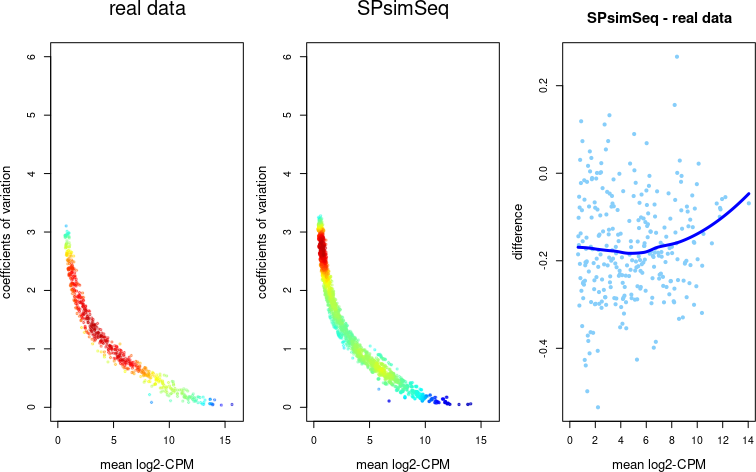Means and zeroes

# compare the relationship between the  mean and fraction of zeros
par(mfrow=c(1,3), mar=c(4,4,4,1))
heatscatter(rowMeans(Y0.log.cpm), rowZeroFrac(Y0.log.cpm), ylim=c(0, 1),
xlim=c(0, 16), colpal="bl2gr2rd", main="real data", xlab="mean log2-CPM",
ylab="fraction of zero counts", cexplot=0.5, alpha = 60, cex.lab=1.25)
heatscatter(rowMeans(Y1.log.cpm), rowZeroFrac(Y1.log.cpm), ylim=c(0, 1),
xlim=c(0, 16), main="SPsimSeq", xlab="mean log2-CPM",
ylab="fraction of zero counts",  cexplot=0.5, alpha = 60,
colpal="bl2gr2rd", cex.lab=1.25)
n.gride <- 1000
min.g   <- seq(0, 20, length.out = n.gride+1)[-n.gride]
max.g   <- seq(0, 20, length.out = n.gride+1)[-1]
mid.g   <- (min.g+max.g)/2
f.real  <- vapply(seq_len(n.gride), FUN.VALUE = double(1), function(r){
x <- Y0.log.cpm[rowMeans(Y0.log.cpm)<=max.g[r] & rowMeans(Y0.log.cpm)>min.g[r],]
y <- ifelse(!is.null(dim(x)), mean(rowZeroFrac(x)), mean(x==0))
y
})
f.SPsim <- vapply(seq_len(n.gride), FUN.VALUE = double(1), function(r){
x <- Y1.log.cpm[rowMeans(Y1.log.cpm)<=max.g[r] & rowMeans(Y1.log.cpm)>min.g[r],]
y <- ifelse(!is.null(dim(x)), mean(rowZeroFrac(x)), mean(x==0))
y
})
sm1 <- loess(I(f.SPsim-f.real)~mid.g)
plot(mid.g, f.SPsim-f.real, xlim=c(0, 14), col="lightskyblue", pch=20, cex.lab=1.25,
cex.main=1.4, main="SPsimSeq - real data", ylab="difference", xlab="mean log2-CPM")
lines(mid.g,predict(sm1, newdata = mid.g), col="blue", lwd=3)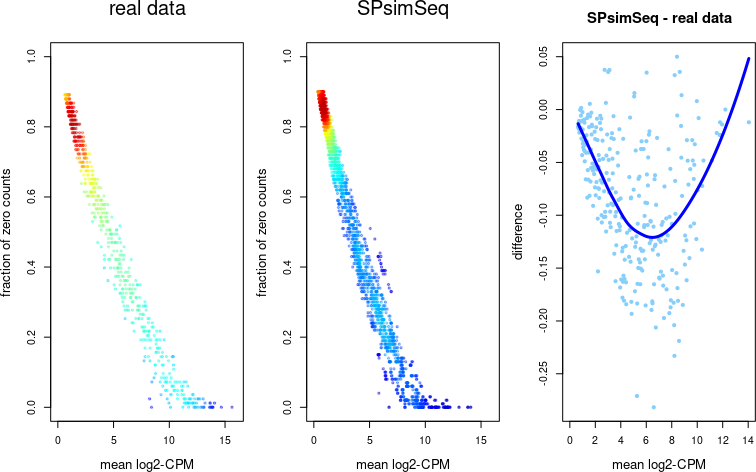Also here we look at the correlation networks

# compare the correlation between genes and samples
Y0.log.cpm2 <- Y0.log.cpm[rowMeans(Y0.log.cpm>0)>0.25, ]
Y1.log.cpm2 <- Y1.log.cpm[rowMeans(Y1.log.cpm>0)>0.25, ]

cor.mat.Y0 <- cor(t(Y0.log.cpm2))
cor.mat.Y1 <- cor(t(Y1.log.cpm2))

cor.vec.Y0 <- cor.mat.Y0[upper.tri(cor.mat.Y0)]
cor.vec.Y1 <- cor.mat.Y1[upper.tri(cor.mat.Y1)]

par(mfrow=c(1,3), mar=c(4,4,3.5,1))
hist(cor.vec.Y0, nclass = 30, probability = TRUE,
border="gray", col="steelblue1", main="real data", xlab="pairwise correlation between genes",
ylim=c(0, 3.5), xlim=c(-1, 1), cex.lab=1.25)
hist(cor.vec.Y1, nclass = 30, probability = TRUE, border="gray",
col="steelblue1",  main="SPsimSeq", xlab="pairwise correlation between genes",
ylim=c(0, 3.5), xlim=c(-1, 1), cex.lab=1.25)

plot(seq(-1, 1, 0.1), seq(-1, 1, 0.1), type="n", xlab="quantile (real data)",
ylab="quantile (simulated data)",  main="correlation quantile-quantile plot")
abline(0, 1, col="gray")
points(quantile(cor.vec.Y0, seq(0, 1, 0.001)), quantile(cor.vec.Y1, seq(0, 1, 0.001)),
col="blue", pch=20, cex=1.5, cex.lab=1.25)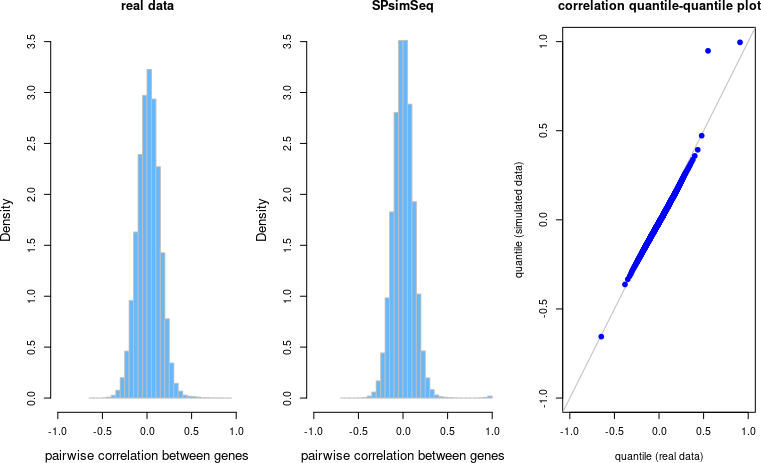sessionInfo()
## R version 4.1.1 (2021-08-10)
## Platform: x86_64-pc-linux-gnu (64-bit)
## Running under: Ubuntu 20.04.3 LTS
##
## Matrix products: default
## BLAS:   /home/biocbuild/bbs-3.14-bioc/R/lib/libRblas.so
## LAPACK: /home/biocbuild/bbs-3.14-bioc/R/lib/libRlapack.so
##
## locale:
##   LC_CTYPE=en_US.UTF-8       LC_NUMERIC=C
##   LC_TIME=en_GB              LC_COLLATE=C
##   LC_MONETARY=en_US.UTF-8    LC_MESSAGES=en_US.UTF-8
##   LC_PAPER=en_US.UTF-8       LC_NAME=C
##  LC_MEASUREMENT=en_US.UTF-8 LC_IDENTIFICATION=C
##
## attached base packages:
##  stats4    stats     graphics  grDevices utils     datasets  methods
##  base
##
## other attached packages:
##   SingleCellExperiment_1.16.0 SummarizedExperiment_1.24.0
##   Biobase_2.54.0              GenomicRanges_1.46.0
##   GenomeInfoDb_1.30.0         IRanges_2.28.0
##   S4Vectors_0.32.0            BiocGenerics_0.40.0
##   MatrixGenerics_1.6.0        matrixStats_0.61.0
##  LSD_4.1-0                   SPsimSeq_1.4.0
##  BiocStyle_2.22.0
##
## loaded via a namespace (and not attached):
##    colorspace_2.0-2       ellipsis_0.3.2         dynamicTreeCut_1.63-1
##    htmlTable_2.3.0        XVector_0.34.0         base64enc_0.1-3
##    rstudioapi_0.13        bit64_4.0.5            AnnotationDbi_1.56.0
##   fansi_0.5.0            mvtnorm_1.1-3          codetools_0.2-18
##   splines_4.1.1          doParallel_1.0.16      cachem_1.0.6
##   Formula_1.2-4          jsonlite_1.7.2         phyloseq_1.38.0
##   WGCNA_1.70-3           cluster_2.1.2          GO.db_3.14.0
##   png_0.1-7              BiocManager_1.30.16    compiler_4.1.1
##   httr_1.4.2             backports_1.2.1        assertthat_0.2.1
##   Matrix_1.3-4           fastmap_1.1.0          limma_3.50.0
##   htmltools_0.5.2        tools_4.1.1            igraph_1.2.7
##   gtable_0.3.0           glue_1.4.2             GenomeInfoDbData_1.2.7
##   reshape2_1.4.4         dplyr_1.0.7            Rcpp_1.0.7
##   jquerylib_0.1.4        rhdf5filters_1.6.0     vctrs_0.3.8
##   Biostrings_2.62.0      multtest_2.50.0        ape_5.5
##   preprocessCore_1.56.0  nlme_3.1-153           iterators_1.0.13
##   xfun_0.27              fastcluster_1.2.3      stringr_1.4.0
##   lifecycle_1.0.1        edgeR_3.36.0           zlibbioc_1.40.0
##   MASS_7.3-54            scales_1.1.1           parallel_4.1.1
##   biomformat_1.22.0      rhdf5_2.38.0           RColorBrewer_1.1-2
##   yaml_2.2.1             memoise_2.0.0          gridExtra_2.3
##   ggplot2_3.3.5          sass_0.4.0             rpart_4.1-15
##   latticeExtra_0.6-29    stringi_1.7.5          RSQLite_2.2.8
##   highr_0.9              foreach_1.5.1          permute_0.9-5
##   checkmate_2.0.0        rlang_0.4.12           pkgconfig_2.0.3
##   bitops_1.0-7           evaluate_0.14          lattice_0.20-45
##   Rhdf5lib_1.16.0        purrr_0.3.4            htmlwidgets_1.5.4
##   bit_4.0.4              tidyselect_1.1.1       plyr_1.8.6
##   magrittr_2.0.1         bookdown_0.24          R6_2.5.1
##   magick_2.7.3           generics_0.1.1         Hmisc_4.6-0
##   DelayedArray_0.20.0    DBI_1.1.1              mgcv_1.8-38
##   pillar_1.6.4           foreign_0.8-81         fitdistrplus_1.1-6
##  survival_3.2-13        KEGGREST_1.34.0        RCurl_1.98-1.5
##  nnet_7.3-16            tibble_3.1.5           crayon_1.4.1
##  utf8_1.2.2             rmarkdown_2.11         jpeg_0.1-9
##  locfit_1.5-9.4         grid_4.1.1             data.table_1.14.2
##  vegan_2.5-7            blob_1.2.2             digest_0.6.28
##  munsell_0.5.0          bslib_0.3.1